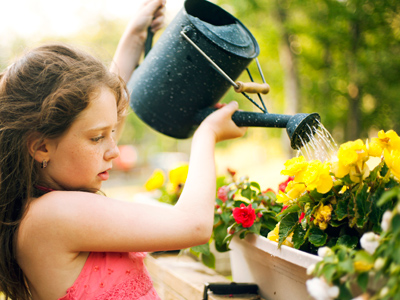If you were investigating the effect different amounts of water had on plant growth, you would need to measure both plant height and amount of water.

# Measurement

Measurement is a vital part of any scientific experiment. This KS2 Science quiz is all about different kinds of measuring equipment, what they are used to measure and the units they use.

Measurement usually involves recording the size or length of something, but almost anything can be measured - electrical current, temperature or force for example. Each of these have their own units - electrical current in amps, temperature in degrees Celsius and force in newtons. Accurate measurement is an essential part of undertaking scientific investigations. You will have used many different types of measuring equipment in your lessons. Measuring beakers, metre wheels and stopwatches - these are all pieces of equipment used to measure things. How many more can you remember? And what is each used to measure?

Check your understanding of measuring equipment and units of measurement with this science quiz.

1.
If you created a catapult and wanted to test how far it could 'throw' different objects, what would you need to measure?
The distance the object is 'thrown' and the height of the catapult
The volume and height of the object
The mass of the objects and the distance each object is 'thrown'
All of the above
Lighter objects would probably be thrown further than heavier ones - why not try this experiment to see if I am right?
2.
To investigate the strength of a magnet, you could see how many paper clips could be suspended from the magnet. What would your units be in this investigation?
Metres
Number of paper clips
Litres
Newtons
Force is measured in newtons, using a forcemetre (or Newtonmeter). In this case, however, the number of paper clips would be the unit of measurement
3.
If you were investigating how hand length is related to height, what measuring equipment would you need?
Weighing scales
A measuring jug and a tape measure
A metre rule and a tape measure
A thermometer
You would need to measure both the length of people's hands and their height
4.
Which unit is a measurement of volume?
Degrees Celsius
Litres
Metres
Amperes
Degrees Celsius measure temperature, metres measure distance and amperes measure electric current
5.
Which units of measurement would you use for the height of an object and the amount of a liquid?
Amps and newtons
Newtons and kilograms
Grams and degrees Celsius
Millimetres and millilitres
Millimetres can measure height and millilitres can measure amounts of liquid
6.
If you were investigating the effect different amounts of water had on plant growth, what would you need to measure?
The plant height only
The amount of water only
Both plant height and amount of water
Neither plant height nor amount of water
As we are looking at the effect different amounts of water have on the height of plants, we need to measure both
7.
Which unit of measurement would you use for length and height?
Degrees Celsius
Litres
Metres
Kilograms
Metres and centimetres are used for measuring length and height
8.
Which unit of measurement would you use for temperature?
Degrees Celsius
Litres
Kilograms
Amperes
Temperature is measured in degrees Celsius or sometimes in degrees Fahrenheit or Kelvin
9.
If you were investigating different materials for insulating coffee, what measuring equipment would you need to test how cool the coffee was after 10 minutes?
A meter rule
A measuring jug
A voltmeter
A thermometer
You would also need a watch or stopwatch to measure the 10 minutes
10.
What measuring equipment would you need to measure the height of an object and the amount of a liquid?
A measuring jug and a ruler
A stopwatch and a measuring jug
A ruler and a thermometer
A forcemeter and a ruler
Measuring jugs measure amounts of liquids and rulers measure length, width or height
You can find more about this topic by visiting BBC Bitesize - How to use scientific equipment

Author:  Sheri Smith